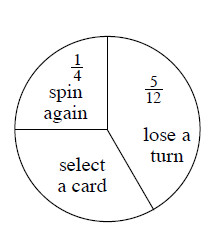### Home > MC2 > Chapter 4 > Lesson 4.2.2 > Problem4-45

4-45.

Darnell designed the spinner below for a game. It still has one incomplete section. .

1. Help him figure out the probability of selecting a card on any turn. Show how you got your answer.

Notice that all three sections make up the entire spinner.
To find the missing area, we must take into account that:
spin again $+$ lose a turn $+$ select a card $= 1$ whole spinner

$\frac{1}{4} + \frac{5}{12} + ? = 1$

Rearrange this equation to solve for the unknown.

$1 - \frac{1}{4} - \frac{5}{12} =\frac{1}{3}$

2. What is the probability that on any turn you will not get to spin again?

What is the probability of spinning again? What is the sum of the probabilities that do not allow spinning again?

$1 −$ the probability of spin again $=$ ?
$1 - \frac{1}{4} = ?$

$\frac{3}{4}$

3. Which is more likely: to lose a turn or to select a card? Show how you know.

What is the probability of losing a turn? What about selecting a card?

$\frac{5}{12} \text{ and } \frac{1}{3}$

Which of these probabilities is higher?Use the eTool below to test the spinner.
Click the link at right for the full version of the eTool: MC2 4-45 HW eTool